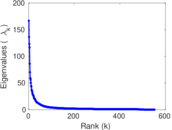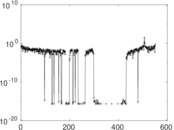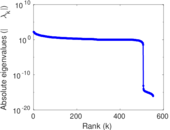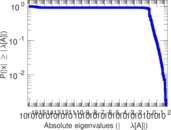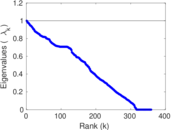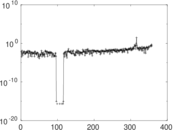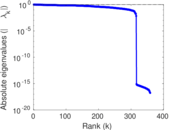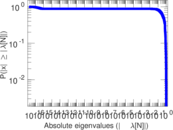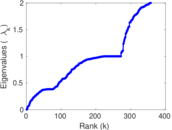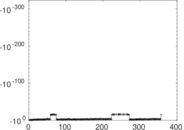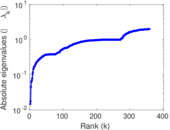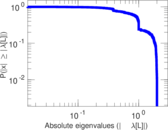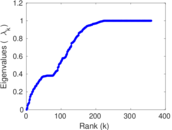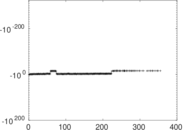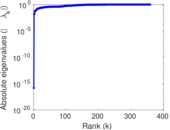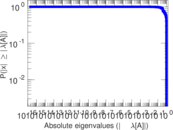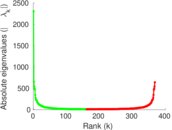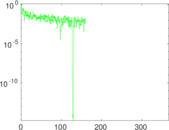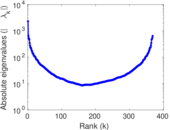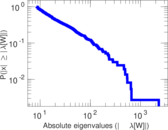# Wikipedia edits (ny)

This is the bipartite edit network of the Nyanja Wikipedia. It contains users and pages from the Nyanja Wikipedia, connected by edit events. Each edge represents an edit. The dataset includes the timestamp of each edit.

 Code `ny` Internal name `edit-nywiki` Name Wikipedia edits (ny) Data source http://dumps.wikimedia.org/ AvailabilityDataset is available for download Consistency checkDataset passed all tests Category Authorship network Dataset timestamp 2017-10-20 Node meaning User, article Edge meaning Edit Network formatBipartite, undirected Edge typeUnweighted, multiple edges Temporal dataEdges are annotated with timestamps

## Statistics

 Size n = 2,338 Left size n1 = 554 Right size n2 = 1,784 Volume m = 11,930 Unique edge count m̿ = 5,097 Wedge count s = 246,794 Claw count z = 11,076,944 Cross count x = 479,617,248 Square count q = 416,557 4-Tour count T4 = 4,331,102 Maximum degree dmax = 1,054 Maximum left degree d1max = 1,054 Maximum right degree d2max = 288 Average degree d = 10.205 3 Average left degree d1 = 21.534 3 Average right degree d2 = 6.687 22 Fill p = 0.005 157 15 Average edge multiplicity m̃ = 2.340 59 Size of LCC N = 1,678 Diameter δ = 14 50-Percentile effective diameter δ0.5 = 4.022 47 90-Percentile effective diameter δ0.9 = 6.353 56 Median distance δM = 5 Mean distance δm = 4.761 60 Gini coefficient G = 0.811 388 Balanced inequality ratio P = 0.157 083 Left balanced inequality ratio P1 = 0.124 308 Right balanced inequality ratio P2 = 0.181 894 Relative edge distribution entropy Her = 0.833 413 Power law exponent γ = 2.640 28 Tail power law exponent γt = 1.961 00 Tail power law exponent with p γ3 = 1.961 00 p-value p = 0.000 00 Left tail power law exponent with p γ3,1 = 1.731 00 Left p-value p1 = 0.028 000 0 Right tail power law exponent with p γ3,2 = 2.071 00 Right p-value p2 = 0.000 00 Degree assortativity ρ = −0.098 886 1 Degree assortativity p-value pρ = 1.489 99 × 10−12 Spectral norm α = 166.763 Algebraic connectivity a = 0.014 783 6 Spectral separation |λ1[A] / λ2[A]| = 1.225 14 Controllability C = 1,197 Relative controllability Cr = 0.541 874

## Plots

### Fruchterman–Reingold graph drawing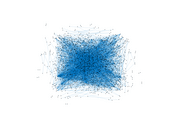### Degree distribution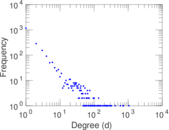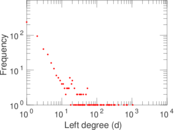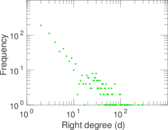### Cumulative degree distribution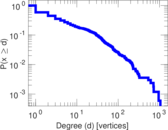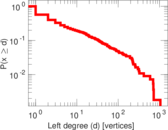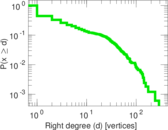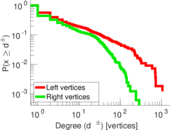### Lorenz curve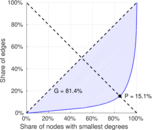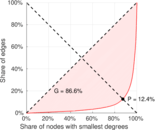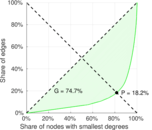### Spectral distribution of the adjacency matrix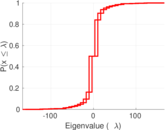### Spectral distribution of the normalized adjacency matrix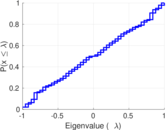### Spectral distribution of the Laplacian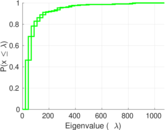### Spectral graph drawing based on the adjacency matrix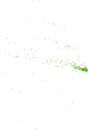### Spectral graph drawing based on the Laplacian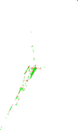### Spectral graph drawing based on the normalized adjacency matrix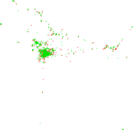### Degree assortativity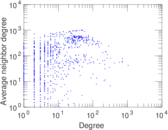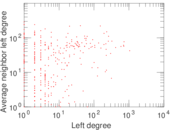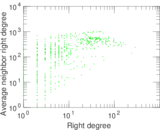### Zipf plot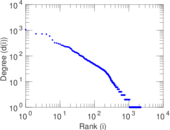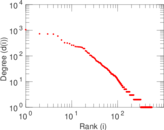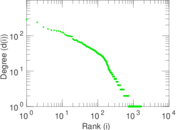### Hop distribution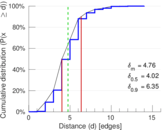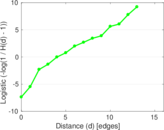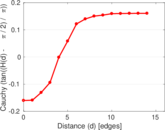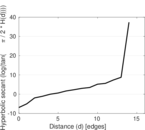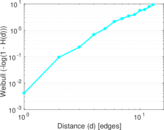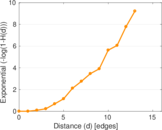### Double Laplacian graph drawing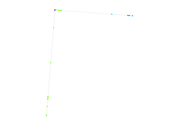### Delaunay graph drawing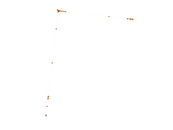### Edge weight/multiplicity distribution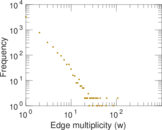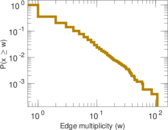### Temporal distribution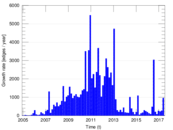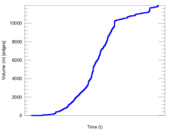### Temporal hop distribution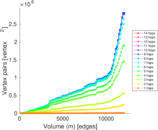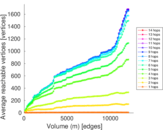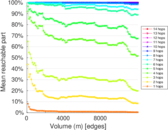### Diameter/density evolution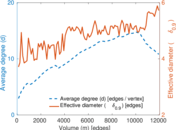### Matrix decompositions plots